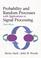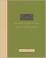### Random Processes in Systems

#### Course Description

The course gives knowledge of the theory of random processes and applications in the systems for signal processing and analysis. Continuous and discrete random signals. Random processes. Stationarity and independence. Correlation functions and power spectral density. Random process in linear systems. Signal parameters estimation. Detection. System identification using cross correlation. Noise modeling and characterization. Noise factor. Optimum linear systems. Wiener filter. Matched filter. Realization of the optimum systems. Signal extraction from the noise by correlation and using matched filter. Signal quantization. Applications in communication, automation and measurements.

#### General Competencies

The course provides fundamental knowledge in stochastic systems and statistical signal processing with emphasis on practical engineering applications. The students will be able to model random processes and deeply understand the concepts of stationarity, ergodicity, independence, correlation and power density, as well as noise modeling concepts. They will be able to design an optimum linear system: Wiener filter or matched filter, or to solve a number of problems in communication, automation, and measurements.

#### Learning Outcomes

1. define and describe concepts of stochastic processes in systems
2. list examples of random signals problems and applications
3. define and explain theory of stochastic theory
4. analyze a practical problem requiring use of stochastic process concept
5. combine acquired knowledge and propose a solution to the given problem
6. evaluate a practical solution obtained using stochastic processes

#### Forms of Teaching

Lectures

12 lectures, preseentation of student team projects

Exams

Midterm and final exam.

Consultations

After lectures

Programming Exercises

Homeworks.

Other Forms of Group and Self Study

Student team project.

Continuous Assessment Exam
Homeworks 50 % 10 % 50 % 0 %
Seminar/Project 50 % 20 % 50 % 0 %
Mid Term Exam: Written 50 % 30 % 0 %
Final Exam: Written 50 % 40 %
Exam: Written 50 % 50 %
Exam: Oral 50 %

#### Week by Week Schedule

1. Course remarks. Introduction to stochastic processes.
2. Stationary and independent stochastic processes.
3. Spectral characteristics of stochastic processes. Examples.
4. Stochastic signals in linear systems. Examples.
5. Stochastic signals in linear systems. Examples of stochastic signals in linear systems. Random process parameter estimation.
6. Signal estimation.
7. Signal detection.
8. Midterm exam.
9. Detection of periodic signals in noise. Noise source modeling. Thermal noise, effective noise temperature, noise in cascade systems.
10. Power spectral density measurement. Bandlimited noise. System identification by crosscorrelation. Examples of power spectral density measurement.
11. Optimal linear systems. Examples of matched and Wiener filters.
12. Scalar quantization. Practical applications of theory: noise in communication systems.
13. Practical applications of theory. Examples: Practical applications of theory.
14. Presentations and discussion of student team projects.
15. Final exam.

#### Study Programmes

Electronic and Computer Engineering (profile)
Theoretical Course (1. semester)
Information Processing (profile)
Theoretical Course (1. semester)
Wireless Technologies (profile)
Theoretical Course (1. semester)

#### LiteratureH. Stark, J. W. Woods (2002.), Probability and Random Processes with Applications to Signal Processing, 3rd Ed., Prentice HallP. Z. Peebles (1993.), Probability, Random Variables, and Random Signal Principles, McGraw-Hill
S. Lončarić, D. Seršić (2011.), Slučajni procesi u sustavima: bilješke s predavanja, FER
S. Lončarić, D. Seršić, T. Petković (2005.), Zbirka zadataka iz slučajnih procesa, FER

#### General

ID 34406
Winter semester
5 ECTS
L2 English Level
L1 e-Learning
45 Lectures